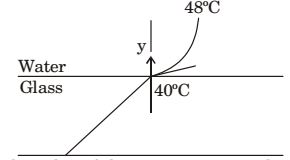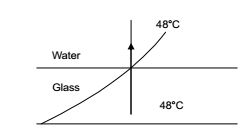## Heat Transfer Miscellaneous

#### Heat-Transfer

1. Grashof number signifies the ratio of

1.  Grashof number = inertia force × Buoyancy force (viscous force)2

##### Correct Option: B

 Grashof number = inertia force × Buoyancy force (viscous force)2

1. The ratio of momentum diffusivity (υ) to thermal diffusivity (α), is called

1.  Pr = μCp = ρVCp = V = V k k k / ρCp α

##### Correct Option: A

 Pr = μCp = ρVCp = V = V k k k / ρCp α

1. Match List-I with List-ll and select the correct answer using the code given below the lists:
 List-I List-II A. Grashof number 1. Mass diffusion B. Schmidt number 2. Transient heat conduction C. Weber number 3. Free convection D. Fourier number 4. Forced convection 5. Surface tension 6. Radiation

1. NA

##### Correct Option: B

NA

Direction: Heat is being transferred by convection from water at 48°C to a glass plate whose surface temp. is exposed to the water is at 40°C. The thermal conductivity of water is 0.6 W/mK and the thermal conductivity of glass is 1.2 W/mK. The spatial gradient of temperature in the water at the water-glass interface is dT/dy = 1 × 104 K/m.1. The heat transfer coefficient h in w/m2K is

1. For convection Heat flux, q = h(Tf – Ti)

 q = kwdTdy w

= 0.6 × 1 × 104 = 6000 W/m
 ∴ h = q = 6000 = 750 W /m2K (Tf – Ti) 8

##### Correct Option: D

For convection Heat flux, q = h(Tf – Ti)

 q = kwdTdy w

= 0.6 × 1 × 104 = 6000 W/m
 ∴ h = q = 6000 = 750 W /m2K (Tf – Ti) 8

1. The value of the temperature gradient in the glass at the water-glass interface in K/m is

1. Temperature of water = 48° C
Temperature of glass plate = 40° C
Thermal conductivity of water = kw = 0.6 W/mK Thermal conductivity of glass = kG = 1.2 W/mK
The spatial gradient of temperature in water at the water-glass interface

 =dT= 1 × 105 K/m dyAt water glass interface
 q = kwdT= kGdTdy w dy G

 ⇒dT= kw ×dTdy G kG dy w

 = 0.6 × 1 × 104 = 0.5 × 104 K/m 1.1

##### Correct Option: C

Temperature of water = 48° C
Temperature of glass plate = 40° C
Thermal conductivity of water = kw = 0.6 W/mK Thermal conductivity of glass = kG = 1.2 W/mK
The spatial gradient of temperature in water at the water-glass interface

 =dT= 1 × 105 K/m dyAt water glass interface
 q = kwdT= kGdTdy w dy G

 ⇒dT= kw ×dTdy G kG dy w

 = 0.6 × 1 × 104 = 0.5 × 104 K/m 1.1